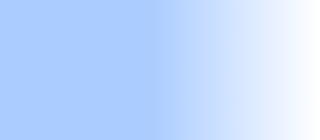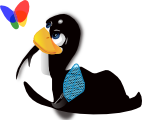# Scratch programming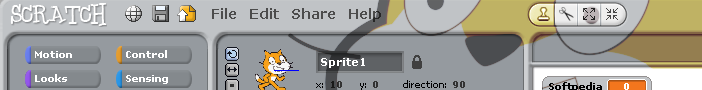### Programming scratch

The Scratch software was launched in 2007. It is free, and is available in nearly 50 languages. The scratch home site has lots of programming examples, many written by young adults, (8 – 16 years) so perhaps the first impression is that Scratch capabilities are limited to that age range. They are not, the Scratch language has a serious side to, as you might expect, since it was developed by the Lifelong Kindergarten Group at the MIT (Massachusetts Institute of Technology) Media Lab. So we all fit into that age range somewhere.

Scratch can be used to develop simple programs, but based on quite advanced concepts. In this respect it provides continuity across the curriculum and beyond. This is perhaps best illustrated by the site This article does not describe how to install Scratch, see the Scratch site itself, or other articles on this site, if installing on a Linux machine

To write a Scratch program simply drag and drop the simple programming fragments to centre column of the screen and connect them together. The fragments, which are a bit like a puzzle pieces, only fit together in a way that makes programming sense. (see screen-shot below). The fragments are colour coded and divided into different logical sections. You should take a minute or two, to look through the different sections. Click on one of the buttons at the top of the left hand column. By default Scratch starts with the motion button and as you can see there are commands to set, go to, move and turn all things you might associate with motion.

Before getting started a word about the stage (drawing area), the coordinates of which are shown below and is reproduced from the Scrath site. Given modern screen resolutions, this is rather small, but adequate, and there is no easy way, that I have found, to change it. So any drawing you do, is restricted to these coordinates.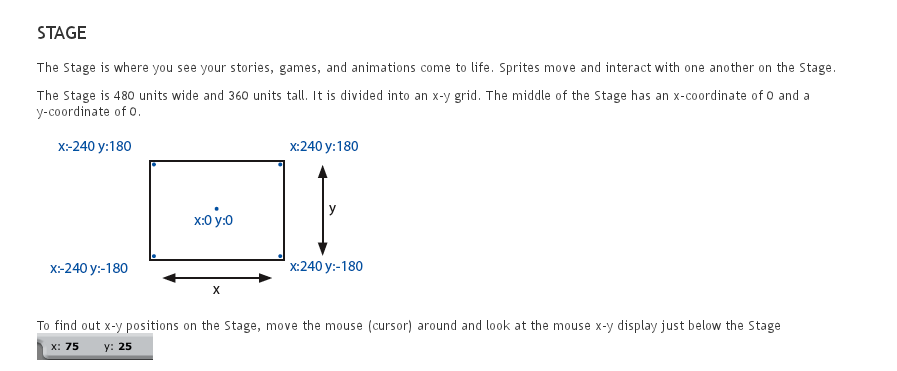You probably don’t want to use the default sprite so right click on the icon (bottom right hand column) and delete it. Next from the centre button (new sprite) select the one you want to use, I’m going to use the pencil.

Not found the sprite you are looking for, then you can draw your own, either with the tool provided, or import your own sprite from your favourite drawing tool.

### A mathematical digression

It’s easy to imagine how to draw a circle with just a peg and length of string. Stick the peg in the ground, attach the string, then. keeping the string taut, walk around the peg once. Easy, but we need to convert that x,y coordinates so a bit of trigonometry is called for.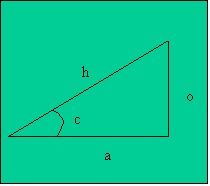From the diagram you can see the y (vertical) coordinate is ‘O’ and the x (horizontal) coordinate is ‘a’. The value ‘h’ represents the radius, and ‘c’ the angle. therefore O = h * sin c and a = cos c. or in our terms y = radius * sin c and x = radius * cos c. That wasn’t to difficult was it?

All we need to do now is change the angle, by a small amount, starting at 0 until we get to 360 degrees, and back to our starting point. The equivalent to walking round the peg.

#### Variables

Back to Scratch. We’ll set the radius to 150 (pixels) and the initial angle to 0. These are our two variables, so select the Variables section and “Make a variable” for angle and radius. Now drag the set option to the centre column and change the drop down box radius, if it isn’t already and set the value to 150. Repeat this of the angle, setting it to 0. Fit the two fragments together.

#### Pen

Next set up the pen. Drag the “clear” option, “pen up”, “set pen size to” to the centre column and fit them together with the other two “set” fragments. Your program should look like this.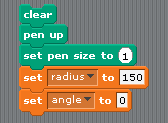While in the section, drag the “pen down” option to the centre column as well because we’ll need it in a shortly.

#### Motion

The next step is to introduce some motion. Go to the motion section and drag two copies of the “go to x: y: fragment” to the centre. We’ll use one to move the pen (sprite) to its starting point. Now you could just set the x and y values to 150 (the radius) and 0, but when programming if you can use variables instead. So go to the variables section, drag a copy of radius and fit it into the position for the x value. Now the pen is in position attach the “pen down” fragment(ready to draw). Everything is now “set up” and ready to go.

In programming terms this is referred to as “setting up the initial conditions”, or simply, “initialisation”.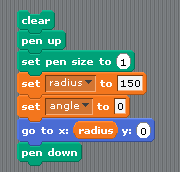#### Operators

Now you have seen how to attach variables to the “set x: y:” fragment, we can extend this idea to set up the equations we are going to need using operators. As we have already seen, the formulae for x and y are

```    x = radius * cos angle
y = radius * sin angle
```

Go to the operators section, and grab, two of the multiplication fragments (top row in diagram below) and two of the “sqrt of 10” fragments. Then go to the variables section and grab two copies of radius and two copies of angle.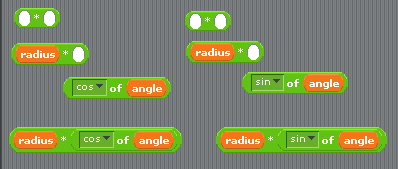Attach the radius variables to the two multiplication fragments (second row) and “multiply” the radius fragment by the cos fragment. Do the same the sin fragment; (row 4).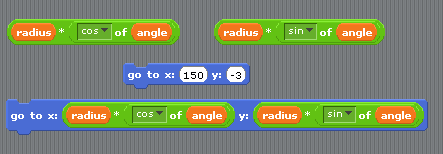These two fragments can now be attached to the other “go to x: y:£ fragment we fetched earlier.This is the formula we need to plot the circle.

#### Control

In this final stage of development we need a way to change the angle from 0 to 360 degrees in order to draw the circle. To do this we’ll use the control fragment “forever if” and another “set angle to ” from the variables section. This will determine just how much to increment the angle. Then select the less than (<) operator and the plus (+) operator from the operators section.
By now you should know how these fragments fit together so they look like the result below.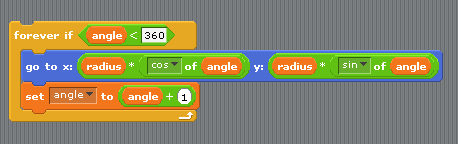#### How does the “forever if” fragment work?

This deserves a little explanation. I could have simply chosen the “forever” fragment and gone round and round, well forever. (or at least until the angle variable gets so big, it breaks the limit on your PC). Instead I choose the “forever if” so I could stop the program after one revolution. This is achieved by setting a condition so the program keeps looping until the angle is greater than (or equal to) 360 degrees. The arrow at the bottom right hand corner, indicates that when the program gets to the end it loops back to the start of the “forever if” fragment. Before going round again I must change the angle. If I forget to do this, and its very easy to forget(!), then the condition, angle < 360 (degrees) never changes and so I go round the loop forever, but never move from the spot; because x and y never change.

#### Running the program

To kick things off, select the “when clicked” fragment and attach it to the start of the program. The complete program is shown below. The program is “run” by clicking on the green flag, either at the top of the program, or the green flag at the top of the stage. It can be stopped by clicking on the red circle above the stage.

At this point save your finished program.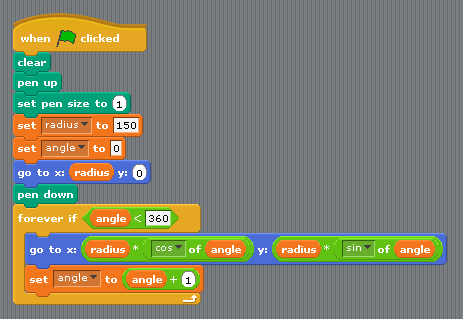### Variations on a theme – lets play

Now that we have a basic program, lets explore. Instead of a constant radius, what happens if the radius changes as we go round? With just a few tweaks lets see. You can imagine the string wrapped around the peg, so that as you walk around the peg it unwraps, getting longer and longer.

#### Archimedes spiral

Most of the program is the same. I’ve added two new variables, rotation and archimedes. The latter determines by how much the radius is changed as the angle is incremented. The rotation variable is set to 6 and archimedes is set to 0.4. You can of course choose different values, just be careful they don’t stray of the stage. The complete program and result is shown below. Save this program to, giving it a different name.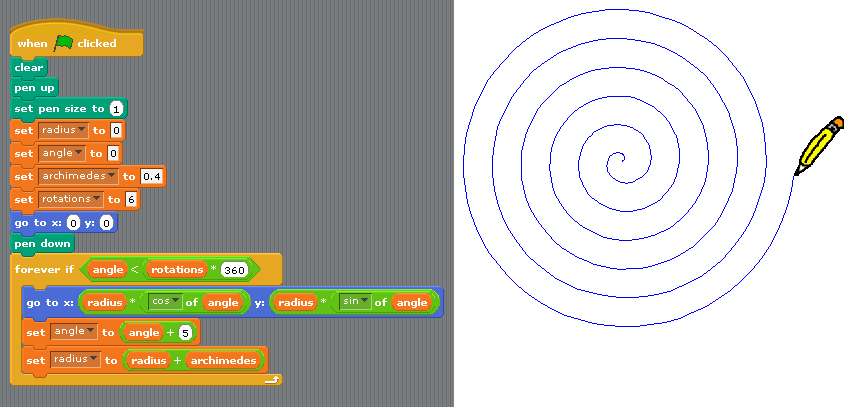#### Ellipse

Go back to the circle program. Instead of a fixed radius suppose the values of x and y are different. Just replace the variable radius with two new variables A and B and see. This is an even simpler change than before, and you can change the shape of the ellipse depending on the values you set A and B to.

You can draw an ellipse with two pegs, and instead of having one centre, move the pegs apart so you have two centres. (A mathematician would call each centre a focus). The two ends of the string are attached to each of the pegs, and as before, walk round the pegs keeping the string taut. Note: As you go round you will have to let the string slip through your hands; because the distance from each peg changes, but the overall length of the string is fixed.

```    x = A * cos angle
y = B * sin angle
```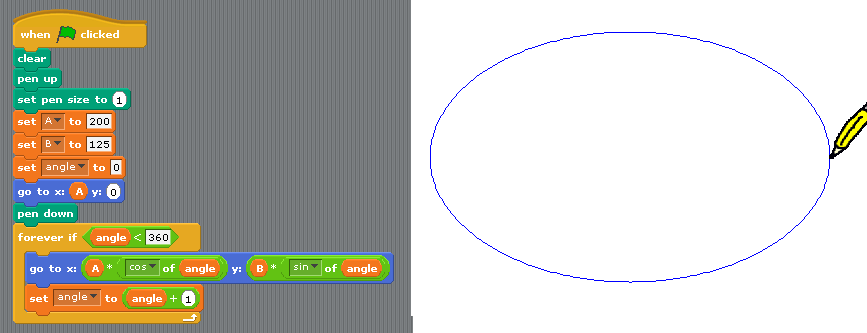#### Lissajous figures

Now that you have seen the effect of using different values for A and B, there is one other variable we can change between the x and y axis and that is the angle. In this case I’ve replaced the variable angle, with two new variables angle1 and angle2 and used a ratio t relate them. In the diagram below the ratio is 2:1. i.e. angle2 is going round twice as fast as angle1.

```    x = A * cos angle1
y = B * sin angle2
```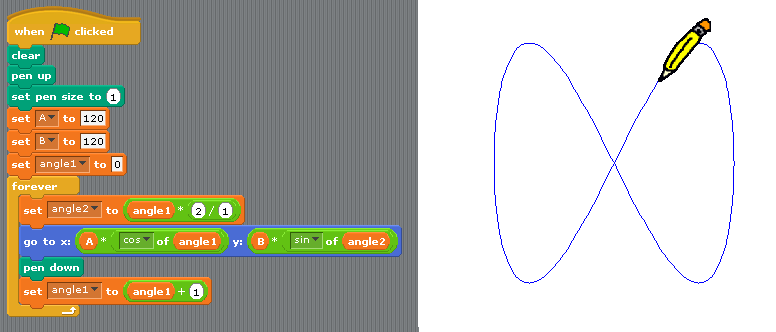In fact the neat thing about Lissajous figures is what happens when you change this ratio. So as well as the ratio 2:1 here are some more examples, 2:3, 4:3, 2,5, 4,5 and 6:7, only I’m afraid I’ve jumbled them up, so they are not in that order. To find out which shape goes with which ratio you’ll just have to plot them and see!!

What shape to I get if A = B and the ratio is set to 1:1 so angle1 = angle2?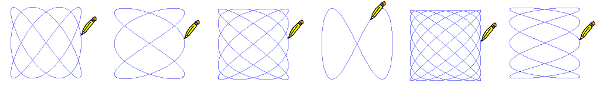#### Who was Lissajous?

Jules Antoine Lissajous, to give him his full name, was a French mathematician who studied this generic family of curves in 1857. A Lissajous curve (or Lissajous figure) is the graph of a system of parametric equations which describe complex harmonic motion

### Summary

I hope you have enjoyed this little excursion into Scratch programming, and maybe learned something along the way. Even with a limited set of program fragments, there is plenty of scope to explore, for all age ranges, so is well worth a look, and it can be installed on both Linux, Windows and Apple machines.

The program examples above, are not intended to be exemplars of good Scratch coding, doubtless someone out there, can improved upon them. The point is simply to show what can be done.

All (functional) program languages are based on sequence, selection (if … else) and repetition, e.g.(loop) forever. so its not difficult to tease out these concepts from Scratch. What this language doesn’t bore you with is the programming language syntax, so is a major simplification which is especially helpful when first introducing children to programming.

The operators section includes the basic arithmetic operations, of add, subtract, multiply and division as well as the logic operators and, or and not. The latter being the fundamental building blocks of any digital computer.

#### How might you progress to one of the mainstream languages?

If you are looking for a deeper understanding of programming, then probably, as a next step I’d recommend is Javascript. This has the advantage of being built into all popular browsers, so is already installed on your PC, and is non proprietary, meaning you are not tied to a particular vendors software. Its syntax is very similar to Java, and C++, providing an easy migration path, to these high level languages.

A word of warning. Firefox, Opera and Chrome’s implementation of javascript is pretty much the same, and follow the World Wide Web (W3C – HTML, CSS) and ECMA (Javascript) specifications quite faithfully. Internet Explorer however is different, so is the last browser I would choose to use to learn javascript.

One criticism levelled, not just of Javascript, but HTML as well, was the ability to produce drawings, graphs etc. Flash used to be the answer, but technology has moved on. Modern browsers now make it possible to render data using a variety of drawing technologies, such as VML, SVG and Canvas. The latter being part of the HTML5 specification, which the W3C (World Wide Web Consortium) plans to finalise by July 2014.

For the purpose of comparison, the following javascript snippet will draw circles and ellipses. It has been deliberately written to look similar to the scratch program structure; and consequently is almost certainly not the best way to program this in javascript. Even if you are not familiar with javascript syntax, hopefully you can spot the similarities with the Scratch program.

Because the <canvas> element is still relatively new, the script will not work in older browsers (e.g. Internet Explorer versions below IE9). Make sure the DOCTYPE at the top of the HTML page is set to <!DOCTYPE html>

To set up a canvas, the same size as the scratch page and optionally add a border, add the element

``` <canvas id="canvas" width="480" height="360" style="border:1px solid black">
```

Other differences to note, when compared to Scratch, are the coordinate system, and in javascript (Java and C++), angles are measured in radians, not degrees.

```

function drawCircle()
{
// Create a circle or ellipse
var canvas = document.getElementById("canvas");
var cntx = canvas.getContext("2d");
cntx.strokeStyle = "#000";            //set pen colour
cntx.beginPath();                     //start new path
var A = 200; var B = 120;             //values for major and minor axis
var originx = 240; var originy = 180; //set origin
cntx.moveTo(originx + A,originy);     //move to starting point
for(var angle=0;angle<2*Math.PI;angle+=0.1)
{
var x = A * Math.cos(angle) + originx
var y = B * Math.sin(angle) + originy;
cntx.lineTo(x,y);                  //draw line to new point
}
cntx.closePath();
cntx.stroke();                       //create stroke
}

```